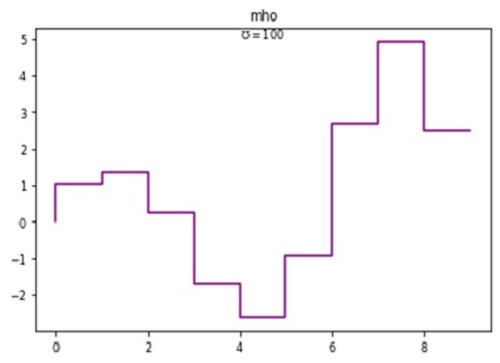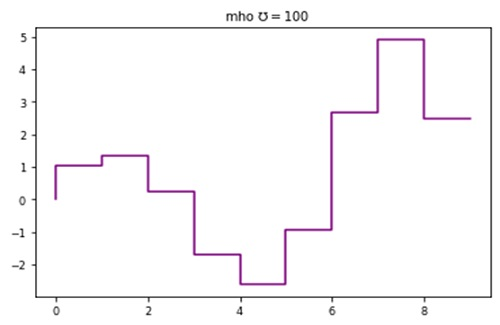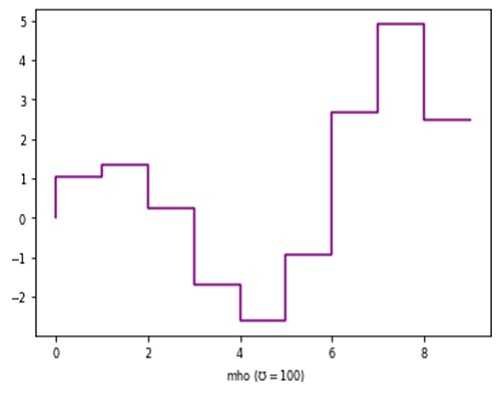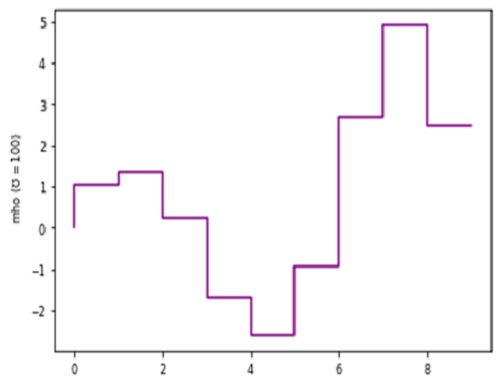# Python | Adding mho in Plot Label

Here, we are going to learn how to add mho in Plot Label?
Submitted by Anuj Singh, on August 07, 2020

℧ mho is very often used greek mathematical letters. They are mostly used in physics, chemistry, fluid dynamics, and other mathematical expressions when there is topic related change (for example resistance, energy change, fluid density) and therefore, they have registered importance in languages.

Therefore, matplotlib has defined a command for usage.

mho

```plt.text(3, 0.4, r'\$\mho=100\$')
``````plt.title(‘mho 'r'\$\mho=100\$')
#Adding ℧ in title of the figure
``````plt.xlabel('mho ('r'\$\mho=100)\$')
#Adding ℧ in x axis label of the figure
``````plt.ylabel('mho ('r'\$\mho=100)\$')
#Adding ℧ in y axis label of the figure
```## Python code for adding mho in plot label

```import numpy as np
import matplotlib.pyplot as plt

x = np.arange(10)
y = np.sin(x)*np.exp(x/5)

# mho
# In text
plt.figure()
plt.plot(x,y, ls='steps', color=  'purple')
plt.title('mho')
plt.text(4, 5, r'\$\mho=100\$')
plt.show()

# In title
plt.figure()
plt.plot(x,y, ls='steps', color=  'purple')
plt.title('  mho 'r'\$\mho=100\$')
plt.show()

# In x-axis label
plt.figure()
plt.plot(x,y, ls='steps', color=  'purple')
plt.xlabel('  mho ('r'\$\mho=100)\$')
plt.show()

# In y-axis label
plt.figure()
plt.plot(x,y, ls='steps', color=  'purple')
plt.ylabel('  mho ('r'\$\mho=100)\$')
plt.show()
```

Output:

```Output is as Figure
```